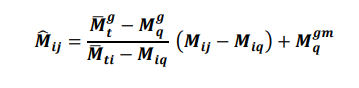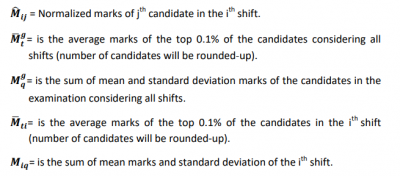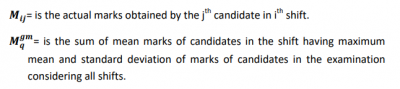# IBPS Marks Normalization 2020 – Process of Normalization in IBPS ExamsIBPS Marks Normalization: Institute of Banking Personnel has conducted the IBPS Clerk Prelims Exam on December 7 & 8, 2019. At the same time, IBPS is about to release IBPS Clerk Prelims Result on 01st January 2020.

Institute of Banking Personnel Selection calculates the marks through the Normalization of the score. IBPS Marks Normalization is done in all its exams from IBPS PO, IBPS Clerk, and IBPS RRB.

## What is IBPS Marks Normalization?

IBPS calculates the marks of all candidates appearing for IBPS Clerk Exam through normalized score calculation. As both the prelims and mains exam is conducted in multiple shifts with varying difficulty levels, Normalization helps in balancing the scores measured on different scales (difficulty shifts ) to a common scale.

### Why is IBPS Normalization Done?

IBPS uses the method of Normalization to convert all marks into a standardized format to make it fair for all candidates appearing for the exam. It is done to evaluate the performance of all the candidates on similar exam parameters i.e. it aims to adjust the difficulty level across different shifts of the exam.

• The normalization is done following the fundamental assumption that in all multi-shifts examination, the distribution of abilities of candidates is the same across all shifts.
• This fundamental assumption holds true since the number of candidates appearing in the exam conducted by the Institute of Banking Personnel Selection is large shift allocation is done at random.

### How is IBPS Marks Normalization Calculated?

The scores calculated using the normalized formula can be corrected up to 5 places of decimal. Given below is the formula for calculation of final scores of candidates in IBPS exam:###Example for IBPS Marks Normalization

The Normalization of Marks is done in the following manner.

1. A candidate got 142 marks on 11 August, 2nd shift.
2. The Average marks of top 0.1% candidates in all sessions = 191
3. Sum of Mean and SD (cut-off) for all sessions = 131
4. Average marks of top 0.1% candidates in 11th August 2nd shift = 185
5. Sum of Mean and SD (which is basically a cut-off)for 11th august 2nd session = 129
6. Normalization marks = (60/56) (13) + 129 = 142.92

The Note in the Official IBPS notification is as follows: Process of Normalization of Score in IBPS Exams

• The corrected scores obtained by each of the candidates in different sessions (if held) will be normalized using the Equipercentile method.

• Scores up to two decimal points shall be taken for the purpose of calculations

‘Normalization’ of scores is commonly found in most banking exams.

Does IBPS PO 2020 Exam have Normalization?
Yes, IBPS PO 2020 Exam has a Normalization procedure. To make the selection process fair, IBPS Marks Normalization is practiced.
What is the Normalization in IBPS Clerk 2020?
Normalization is a process to adjust values measured on different scales to a notionally common scale. It is done to evaluate the performance of the candidates on the basis of similar exam parameters and aims to adjust the difficulty level across different shifts of the exam
Does Accuracy matter in Normalization?
There is no such differential marking scheme based on accuracy. The normalized mark is based on – toppers marks, average marks, and standard deviation. So, the candidate’s final mark is all that matters.
Can marks get reduced because of IBPS PO Normalization process?
Normalization of marks means increasing or decreasing the marks obtained by students in different timing sessions to a certain number. Similarly, students who have scored 90 marks will also be marked as 60 marks following the normalization process
What is Normalization formula?
The Normalization formula is as provided above in this page. The Normalization formula is common for most competitive government exams.
How is Normalization Done?
Normalization is a process to adjust values measured on different scales to a notionally common scale. It is done to evaluate the performance of the candidates on the basis of similar exam parameters and aims to adjust the difficulty level across different shifts of the exam